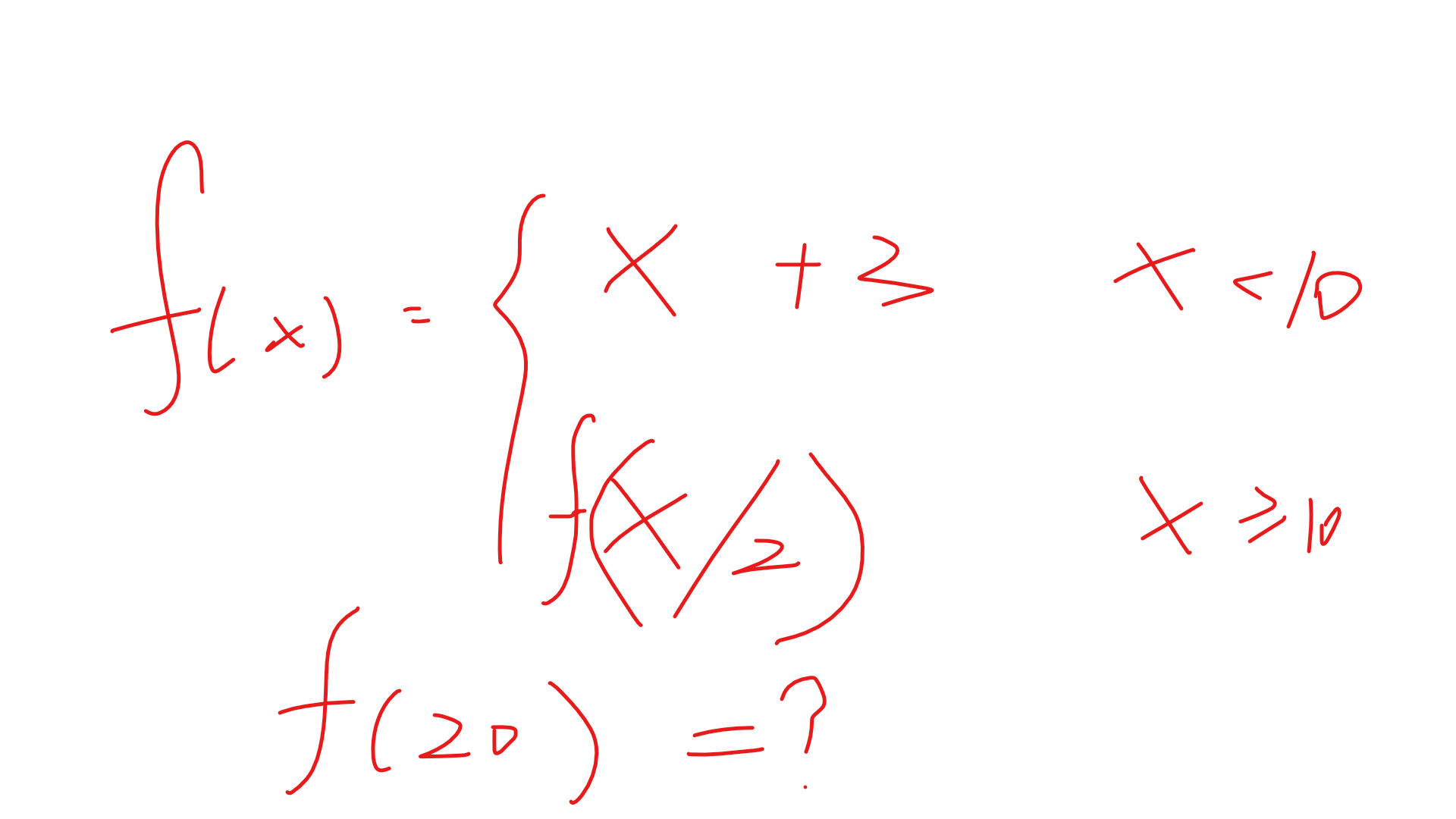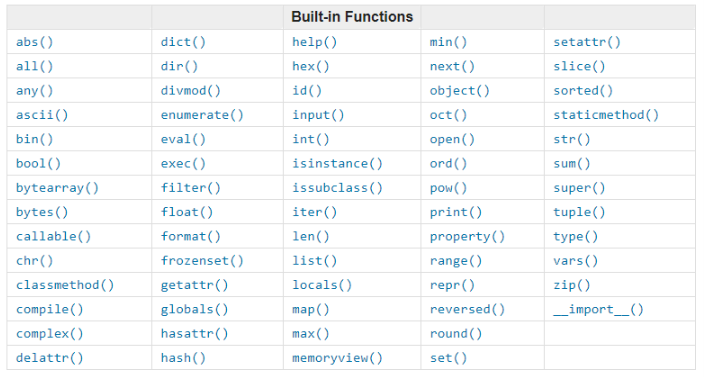# #12 Python函数

## 前言

• 大大减少重复代码

• 可以重复使用

• 程序具有扩展性

### 二、函数初始化

In : def func():         # func是函数名，可随意更改
...:     print('A')      # 函数的内容
...:     print('B')
...:     print('C')

• 函数初始化以关键字 def 开头，之后接函数名和一对圆括号，再加一个冒号

•  函数的内容使用缩进

In : def func():
...:     '''
...:     function's description      # 三引号将函数的说明引起来
...:     '''
...:     pass

### 三、函数返回与调用

In : def add():
...:     '''
...:     calculate a plus b
...:     '''
...:     a = 5
...:     b = 6
...:     c = a + b
...:     return c              # 将结果c返回

In : def tell_name():
...:     '''
...:     '''
...:     print('I am MinuteSheep')

In : tell_name()       # 调用函数
I am MinuteSheepIn : def func(x):
...:     if x < 10:
...:         ans = x + 3
...:         print(ans)
...:         return ans
...:     else:
...:         return func(x/2)    # 递归调用自己
...:

In : func(20)
8.0
Out: 8.0

函数递归的规则：

• 必须有一个明确的结束条件

• 每次进入递归后，运算规模比上一次小（否则无限大，问题无法求解，内存会被占满）

### 四、函数参数

In : def add(x,y):       # 函数拥有参数时，将其写在括号里即可
...:     '''
...:     calculate x plus y
...:     '''
...:     return x + y    # return语句可以返回计算式

Out: 6

In : def add(x,y):      # x，y就是形参，只是一个名称而已，并不占用内存空间
...:     '''
...:     calculate x plus y
...:     '''
...:     return x + y

In : add(1,5)           # 1，5是实参，本质为 在调用函数时，将 x=1，y=5传给add函数，相当于两变量，占用内存资源
Out: 6

In : def print_num(a,b,c):
...: '''
...: print num of nums
...: '''
...: print(a)
...: print(b)
...: print(c)

In : print_num(1,3,5)

1
3
5

# 可以看到1和a对应，3和b对应，5和c对应，这就是按位置参数调用

In : def print_num(a,b,c):
...:     '''
...:     print num of nums
...:     '''
...:     print(a)
...:     print(b)
...:     print(c)
...:

In : print_num(b=7,a=1,c=10)
1
7
10

# 直接给变量赋值，必须指定是哪个参数

In : def print_num(a,b,c):
...:     '''
...:     print num of nums
...:     '''
...:     print(a)
...:     print(b)
...:     print(c)
...:

In : print_num(5,c=1,b=10)    # 混合使用方法
5
10
1

In : print_num(a=4,6,c=10)   # 混合使用时，位置参数必须要在关键参数之前，否则报错
File "<ipython-input-13-99c9e145d0a7>", line 1
print_num(a=4,6,c=10)
^
SyntaxError: positional argument follows keyword argument

In : def print_num(a,b,c=5):   # 指定c的默认值为5
...:     print(a)
...:     print(b)
...:     print(c)

In : print_num(1,2)       # 使用默认参数
1
2
5

In : print_num(1,2,3)     # 按位置参数，令c=3
1
2
3

In : print_num(1,2,c=3)   # 按关键参数，令c=3
1
2
3

In : def print_num(a,b,c):
...:     print(a,b,c)
...:

In : print_num(1,2,3,4)   # 当参入的参数多于给定的参数时，会抛出异常
---------------------------------------------------------------------------
TypeError                                 Traceback (most recent call last)
<ipython-input-23-a8e0af192084> in <module>
----> 1 print_num(1,2,3,4)

TypeError: print_num() takes 3 positional arguments but 4 were given

*args：将传入的多余参数构造成一个元组

In : def print_num(a,b,c,*args):
...:     print(a,b,c)
...:     print(args)
...:

In : print_num(1,2,3,4,5,6,7,8,9,0)    # 多余的参数变成元组保存下来
1 2 3
(4, 5, 6, 7, 8, 9, 0)

**kwargs：将传入的多余参数构造成一个字典

In : def print_num(a,b,c,**kwargs):
...:     print(a,b,c)
...:     print(kwargs)
...:

In : print_num(1,2,3,f=3,g=45,y=123)     # 将多余的参数构造成字典保存下来
1 2 3
{'g': 45, 'y': 123, 'f': 3}

In : def print_num(a,b,c,*args,**kwargs):
...:     print(a,b,c)
...:     print(args)
...:     print(kwargs)
...:

In : print_num(1,2,3,4,4,5,f=45,g=23,u=345)
1 2 3
(4, 4, 5)
{'g': 23, 'u': 345, 'f': 45}

### 五、函数变量

 1 quan_jv_bian_liang = 555
2
3
4 def func():
5     jv_bu_bian_liang = 666
6     ans = quan_jv_bian_liang + jv_bu_bian_liang
7     print(ans)
8
9
10 print(quan_jv_bian_liang)   # 访问全局变量
11
12 print(jv_bu_bian_liang)     # 访问局部变量

E:\PythonProjects\跟着MS学Python>python -u "e:\PythonProjects\跟着MS学Python\#12\1.py"
555                                  # 全局变量能正确访问
Traceback (most recent call last):   # 局部变量不能够访问
File "e:\PythonProjects\跟着MS学Python\#12\1.py", line 12, in <module>
print(jv_bu_bian_liang)
NameError: name 'jv_bu_bian_liang' is not defined

quan_jv_bian_liang = 555

def func():
jv_bu_bian_liang = 666
ans = quan_jv_bian_liang + jv_bu_bian_liang
print(ans)

func()

# 运行结果
E:\PythonProjects\跟着MS学Python>python -u "e:\PythonProjects\跟着MS学Python\#12\1.py"
1221# 可以看到全局变量在函数中可以被正确访问

quan_jv_bian_liang = 555

def func():
jv_bu_bian_liang = 666
quan_jv_bian_liang = 777    # 修改全局变量的值
ans = quan_jv_bian_liang + jv_bu_bian_liang
print(ans)

func()
print(quan_jv_bian_liang)

# 运行结果
E:\PythonProjects\跟着MS学Python>python -u "e:\PythonProjects\跟着MS学Python\#12\1.py"
1443   # 在函数内，全局变量被修改
555    # 在主程序中，全局变量并没有被修改

quan_jv_bian_liang = 555

def func():
jv_bu_bian_liang = 666
global quan_jv_bian_liang   # 申明这是全局变量即可
quan_jv_bian_liang = 777
ans = quan_jv_bian_liang + jv_bu_bian_liang
print(ans)

func()
print(quan_jv_bian_liang)

# 运行结果
E:\PythonProjects\跟着MS学Python>python -u "e:\PythonProjects\跟着MS学Python\#12\1.py"
1443
777     # 被正确更改# 尽量不要在函数内更改全部变量，这样做可能会导致变量使用混乱，让自己使用变量出错

### 六、高阶函数

In : def dunc(x):
...:     return x*x
...:

In : def add(a,b,f):        # 将函数作为参数
...:     print(f(a) + f(b))

5

### 七、匿名函数

In : def func(x):
...:     return x*x

# 上面的函数使用下面的匿名函数代替：

In : func = lambda x:x*x

In : func(10)
Out: 100

### 八、内置函数posted @ 2019-01-30 15:55  MinuteSheep  阅读(585)  评论(0编辑  收藏  举报